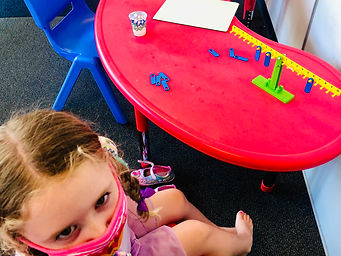## Ms. AJ

### Target 1​

###### Lesson Type:

Continuation

Algebra

:

Variable

Determine the unknown number in an informal addition or subtraction problem.

###### 1:

Use informal understandings of addition and subtraction to solve problems where one addend or subtrahend is given, and the sum or difference is given to solve for the unknown number.

1st

###### Vocabulary:

Unknown, Missing Number, Key

Activities:

Students solved "Missing Number" problems in which they tried to determine an unknown number of objects in a visual addition equation (using counting toys).

Students solved "Missing Number" problems in which they tried to determine an unknown number of objects in a visual subtraction equation (using counting toys).

As an added challenge, students solved missing number problems by using a shape key, where each shape magnet represents a number.

Students used number balances to try to find different ways to balance a given number on one side with two or more numbers on another side.### Home Exploration

###### Guiding Questions:## Absent Students:

Jasmine

### Target 2

:

###### 1:

Use the given information in a problem to identify the correct answer.

###### 2:

Use the given information to determine which is not the correct answer.

###### 3:

Think outside of the box to solve problems.

1st

###### Vocabulary:

Clue, Information

Activities:

Students reviewed “process of elimination”-type puzzles, in which they were given clues as to figure out which pieces of information fit together, and which do not. They learned to eliminate the impossible in order to help them find the answers.### Home Exploration

###### Guiding Questions:### Target 3

:

###### Vocabulary:

Activities:### Home Exploration